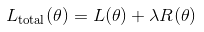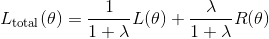# How to balance different loss term?

For example,
The network has two outputs, ouput1 is from an intermedia layer and output2 is from the last layer.
And my total loss:
`loss_total = loss_1 + loss_2`
where loss_1 is calculated using output1 and loss_2 is calculated using output2.
Now the problem is the loss_total seems to be dominated by loss_1 , and loss_2 doesn’t play a role。
I try to put some weights on loss_1, loss_2, like:
`loss_total = loss_1 * 0.001 + loss_2 *0.01`
However, this is not likely to work.
Does any one has some ideals on the similar problems?

I have no idea which specific losses you are using. Anyway, the following may help solving your problem.
Just define your `loss_total` as:

``````loss_total = alpha*loss_1 + (1-alpha)*loss_2
``````

and finetune the hyperparameter `alpha` in the interval (0,1).

If you say that `loss_1` dominates the `total_loss`, then you’ll have o choose an `alpha` that is closer to 0 than to 1. Maybe you can start with `alpha = 0.4` and, if it still does not work, decrease it by a fixed step of 0.05 or so, until you get reasonable results.

However, before doing this cross-validation, you may wish to try training with `alpha = 0`, just to make sure that you can in fact minimize `loss_2`. There could be a bug in the definition of `loss_2` and this procedure allows you to check that.

Hi @dpernes. I have met the same problem as above and appreciate your solution. And I hope the network can learn the alpha itself just like other hyperparameters of the network. But the parameters in the network are optimized by the optimizer like SGD. So how can I do to optimize the alpha automaticly ?

Hi @Pongroc.

In principle, `alpha` is a hyperparameter, so it is not learned by gradient-based optimization, but rather by cross-validation. (You know what cross-validation is, right?)

You may ask why is that so - and that’s precisely what I am going to try to explain. Suppose that `loss_1` is typically much larger than `loss_2`. Then, if you try to learn `alpha` and the remaining parameters jointly, in such a way that the `loss_total` is minimized, most likely you will get a value for `alpha` that is very close to 0, i.e. the optimization simply removes the contribution of `loss_1` to your `loss_total`. This does not mean, however, that `loss_1` will be low - on the contrary, it will probably be high. If both `loss_1` and `loss_2` are measurements of how well your model behaves (in two different senses), then probably you want both to be relatively low instead of having one that is very low and the other one that is very high. The role of the hyperparameter `alpha` is, therefore, to weight these two losses in some way that optimizes the performance of your model, not necessarily minimizing `loss_total`.

If it makes it easier for you to understand, you may also think of it in the context of regularization. You usually do not learn your regularization hyperparameter together with the model parameters, do you?
Note that the usual regularized loss has a form that is very similar to the loss I proposed:,

whereis some regularization function (e.g. L-2 norm).
Dividing the RHS by a constant (independent of theta) does not change the minimizer of the function, so I may equivalently redefine the loss as:,

which, by setting,

has exactly the same form as the `loss_total` that I proposed in my first reply to this topic.
Now, note that if we set `alpha` to a value that is very close to 0 (or, equivalently, if we set lambda to a very large value), our `loss_total` will be totally dominated by the regularization term, and so we’ll get a model with a very poor performance (high bias), because it basically learns nothing related with the specific problem we are trying to solve. On the other hand, if we set `alpha` to a value that is very close to 1 (or, equivalently, if we set lambda to a very small value), our `loss_total` will be almost unregularized, and so we are likely to have a model that does not generalize well (high variance).